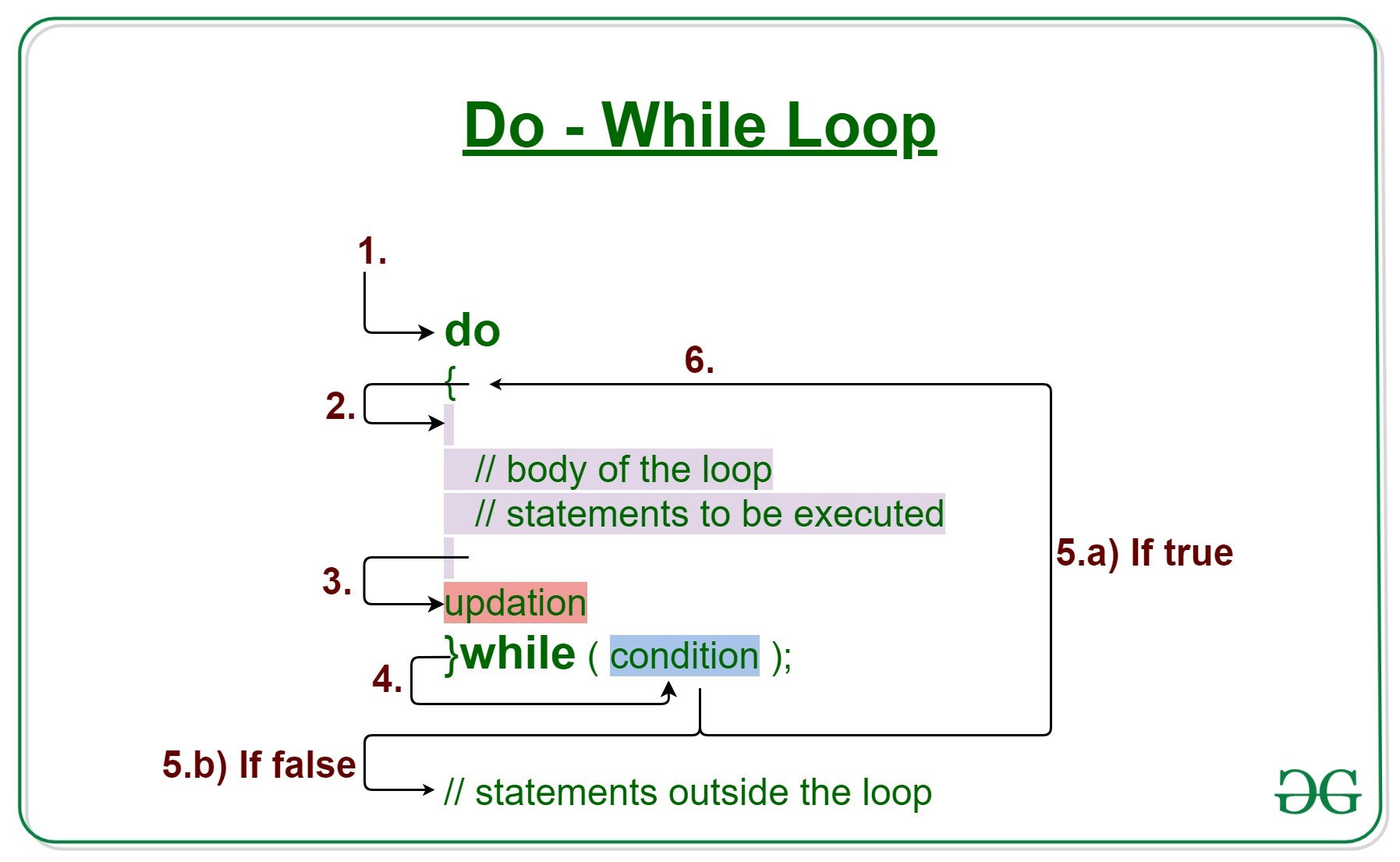Related Articles

# Java do-while loop with Examples

• Difficulty Level : Basic
• Last Updated : 17 Feb, 2021

Loops in Java come into use when we need to repeatedly execute a block of statements.

Java do-while loop is an Exit control loop. Therefore, unlike for or while loop, a do-while check for the condition after executing the statements or the loop body.Syntax:

```do
{
// loop body

update_expression
}
while (test_expression);
```

The various parts of the do-while loop are:

1. Test Expression: In this expression we have to test the condition. If the condition evaluates to true then we will execute the body of the loop and go to update expression. Otherwise, we will exit from the while loop.
Example:
`i <= 10`
2. Update Expression: After executing the loop body, this expression increments/decrements the loop variable by some value.
Example:
`i++;`

How does a do-While loop executes?

1. Control falls into the do-while loop.
2. The statements inside the body of the loop get executed.
3. Updation takes place.
4. The flow jumps to Condition
5. Condition is tested.
1. If Condition yields true, goto Step 6.
2. If Condition yields false, the flow goes outside the loop
6. Flow goes back to Step 2.

Example 1: This program will try to print “Hello World” 5 times.

 `// Java program to illustrate the do-while loop`` ` `class` `dowhileloopDemo {``    ``public` `static` `void` `main(String args[])``    ``{`` ` `        ``// initialisation expression``        ``int` `i = ``1``;``        ``do` `{`` ` `            ``// Print the statement``            ``System.out.println(``"Hello World"``);`` ` `            ``// update expression``            ``i++;``        ``}``        ``// test expression``        ``while` `(i < ``6``);``    ``}``}`
Output:
```Hello World
Hello World
Hello World
Hello World
Hello World
```

Dry-Running Example 1: The program will execute in the following manner.

```1. Program starts.
2. i is initialized with value 1.
3. Execution enters the loop
3.a) "Hello World" gets printed 1st time.
3.b) Updation is done. Now i = 2.
4. Condition is checked. 2 < 6 yields true.
5. Execution enters the loop
5.a) "Hello World" gets printed 2nd time.
5.b) Updation is done. Now i = 3.
6. Condition is checked. 3 < 6 yields true.
7. Execution enters the loop
7.a) "Hello World" gets printed 3rd time
7.b) Updation is done. Now i = 4.
8. Condition is checked. 4 < 6 yields true.
9. Execution enters the loop
9.a) "Hello World" gets printed 4th time
9.b) Updation is done. Now i = 5.
10. Condition is checked. 5 < 6 yields true.
11. Execution enters the loop
11.a) "Hello World" gets printed 5th time
11.b) Updation is done. Now i = 6.
12. Condition is checked. 6 < 6 yields false.
13. The flow goes outside the loop.
```

Example 2:

 `// Java program to illustrate the do-while loop`` ` `class` `dowhileloopDemo {``    ``public` `static` `void` `main(String args[])``    ``{`` ` `        ``int` `x = ``21``, sum = ``0``;`` ` `        ``do` `{`` ` `            ``// The line will be printed even``            ``// if the condition is false``            ``sum += x;``            ``x--;`` ` `        ``} ``while` `(x > ``10``);``        ``System.out.println(``"Summation: "` `+ sum);``    ``}``}`
Output:
```Summation: 176
```

Related Articles:

Attention reader! Don’t stop learning now. Get hold of all the important Java Foundation and Collections concepts with the Fundamentals of Java and Java Collections Course at a student-friendly price and become industry ready. To complete your preparation from learning a language to DS Algo and many more,  please refer Complete Interview Preparation Course.

My Personal Notes arrow_drop_up